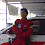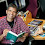## Saturday, April 30, 2011

### Alternatives to the CAPM: Part 4: Market-Implied cost of equity

As you can see from each of the alternatives laid out in the previous three parts, there are assumptions and models underlying each alternative that can make users uncomfortable. So, what if you want to estimate a model-free cost of equity? There is a choice, but it comes with a catch.

To see the choice, assume that you have a stock that has an expected annual dividend of \$3/share next year, with growth at 4% a year and that the stock trades at \$60. Using a very simple dividend discount model, you can back out the cost of equity for this company from the existing stock price:
Value of stock  = Dividends next year / (Cost of equity - growth rate)
\$ 60  = \$3.00/ (Cost of equity -4%)
Cost of equity = 9%
The mechanics of computing implied cost of equity become messier as you go from dividends to estimated cash flows and from stable growth models to high growth models, but the principle remains the same. You can use the current stock price and solve for the cost of equity. For those of you who use Excel, the goal seek function or solver work very well at doing this job, even in the most complicated valuations.

This cost of equity is a market-implied cost of equity. If you are in corporate finance and need a cost of equity to use in your investment decisions, it would suffice. If you were required to value this company, though, using this cost of equity to value the stock would be pointless since you would arrive at a value of \$ 60 and the not-surprising conclusion that the stock is fairly priced.

So, what point is there to computing an implied cost of equity? I see three possibilities.
1. One is to use a conventional cost of equity in the valuation and to compare the market-implied cost of equity to the conventional one to see how much "margin for error" you have in your estimate. Thus, if you find your stock to be undervalued, with an 8% cost of equity, but the implied cost of equity is 8.5%, you may very well decide not to buy the stock because your margin for error is too narrow; with an implied cost of equity of 14%, you may be more comfortable buying the stock. Think of it as a marriage of discounted cash flow valuation with a margin of safety.
2. The second is to compute a market-implied cost of equity for an entire sector sector and to use this cost as the cost of equity for all companies in that sector. Thus, I could compute the implied cost of equity for all banks of 9%, using an index of banking stocks and expected aggregate dividends on that index.  I could then use that 9% cost of equity for any bank that I had to value. This, in effect, brings discounted cash flow valuation closer to relative valuation; after all, when we compare price to book ratios across banks, we are assuming that they all have the same risk (and costs of equity).
3. The third is to compute the market-implied cost of equity for the same company each period for a number of periods and to use that average as the cost of equity when valuing the company now. You are, in effect, assuming that the market prices your stock correctly over time but can be wrong in any given time period.
I use traditional models of risk and return to estimate costs of equity in valuation but I use market-implied costs of equity extensively. As those of you who track my equity risk premium estimates and posts know, I compute an implied equity risk premium for the S&P 500 every month, using exactly the approach described above (though I augment dividends with buybacks). When I value individual companies, I do compare my estimates of cost of equity with the market-implied estimates. Finally, when I am concerned that the beta for a firm is not reflecting its underlying risk, because the sector itself has changed, I compute a market-implied cost of equity for the sector. For instance, after the banking crisis in 2008, I felt that using the beta for a bank or even a sector-average beta to estimate the cost of equity made no sense, since much of the data used in the estimates reflected pre-crisis returns. Consequently, I used the S&P banking index to back out an implied cost of equity (which yielded an estimate almost 4% higher than the CAPM estimate) and used it in my valuations.

The series on alternatives to the CAPM
Alternatives to the CAPM: Part 1: Relative Risk Measures
Alternatives to the CAPM: Part 2: Proxy Models
Alternatives to the CAPM: Part 3: Connecting cost of equity to cost of debt
Alternatives to the CAPM: Part 4: Market-implied costs of equity
Alternatives to the CAPM: Part 5: Risk adjusting the cash flows
Alternatives to the CAPM: Wrapping up

1.Professor,

I am biased towards the camp where I believe ERP calculated from an implied methodology make sense as they are portraying an average of the whole market and an undervalued/overvalued stock is as likely to be factored into the premium.

But I struggle with making intuitive sense of calculating implied cost of equity for an individual stock. They are not predictive or fundamental measures of risk one should assign to a stock.They loose their potential to find under/over valued stocks as they will more or less lead one towards the price the security is currently trading at.

After all goal of any investor is to exploit any inefficiency's that market may be factoring into a stock as measured by it's risk or cost of equity.

Taking GFC example of banking industry, prior to crisis we would have understated the risk in banking stocks with Implied risk premiums, and post crisis one would have overstated the risk in banking stocks as they subsequently became one of the best performers.

I might come across with a circular argument, but thought would lay it out anyways.

Would value your take on it.

Thanks.

2.This actually sounds a lot like reverse-engineered DCF valuation, except that you're using goal seek to find the discount rate implied arbitrarily by the market itself, rather than the growth rate in revenues / net income that would force the PV of future cash flows to equal the stock price...

3.Exactly. It is an IRR of a DCF valuation...

4.Professor, can i compare a market implied CoE with a firm's ROIC to determine EP spread? And hence come to a conclusion on whether growth is value accretive or destructive.

5.This comment has been removed by a blog administrator.

6.This comment has been removed by a blog administrator.

7.Professor,
Can I use it to calculate cost of equity with the historical dividend ? otherwise how to estimate the future divided? pl suggest.. I will be greatful to u.

8.Prof,
How to compute dividend for the next year? ist it estimated(based on the trend in dividend payment) and growth rate( estimated based on the past trend).. pl reply.. It will be useful for my research..

Prof. M S Devi..
msdevi@gmail.com

9.Dear Prof,

How to estimate the future dividend and growth rate? pl suggest.. It will be useful for my research.

Prf. M S Devi
msdevi@gmail.com

10.Dear Prof,
If we use the dividend discount model when used to calculate the cost of equity and an xyz co. pays no cash dividend in that year leading zero payout ratio and zero growth rate resulting in zero cost of equity is that okay?
What will be the cost of equity if an organization pays no cash but only stock dividend in a particular year? Kindly guide about this.

Given the amount of spam that I seem to be attracting, I have turned on comment moderation. I have to okay your comment for it to appear. I apologize for this intermediate oversight, but the legitimate comments are being drowned out by the sales pitches and spam.